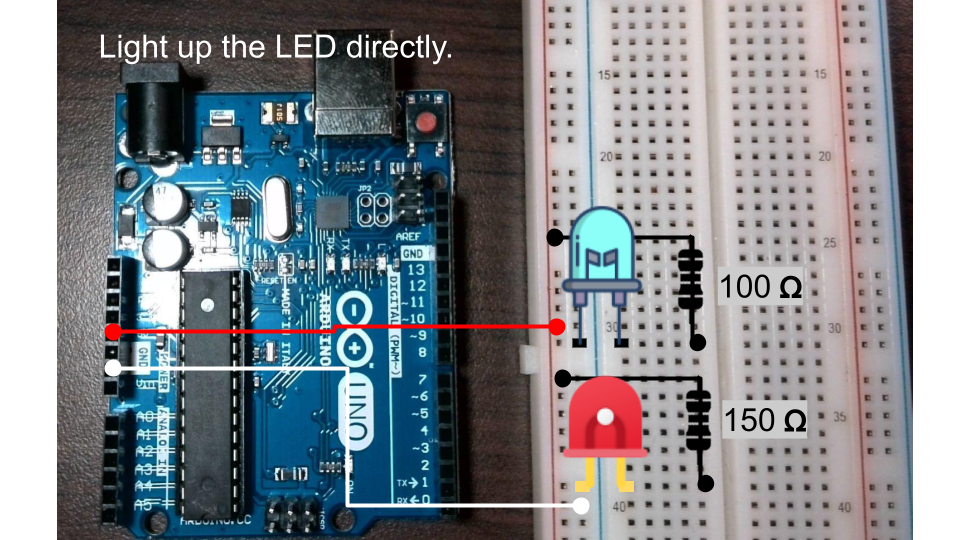Learn how to light up LEDs with an Arduino Uno board and the proper wiring techniques. Discover the voltage requirements for different colored LEDs and how to calculate the necessary resistor value using Ohm’s law. Follow the step-by-step instructions and see how easy it is to connect and control your own red and blue LEDs with an Arduino Uno board.

The Arduino Uno development board provides only 5V or 3.3V of electricity, which is generally too high. To avoid burning out the LED, we must add a resistor.
First of all, you need to understand Ohm’s law and resistor color codes.

Ohm’s Law: The current flowing through a circuit is proportional to the voltage applied and inversely proportional to the resistance in the circuit.

Ohm’s Law formula:

V = IR, voltage = current x resistance

V = voltage in volts I = current in amperes R = resistance in ohms

To calculate the required resistance value, the formula becomes:

R = V / I, resistance = voltage / current

### LED Current

The typical current value for a red LED is around 20mA, and for a blue LED, it is also around 20mA. However, the actual current value may vary depending on the specific LED and circuit configuration. It’s important to check the datasheet or specifications for the LED to determine the appropriate current rating for your circuit.

### LED Voltage

Red LED: 2.1-2.6V
Blue LED: 3.2-4.0V

### Prepare Resistor

RED LED resistor:

R = V / I = (5-2) / 0.02 = 150

You can learn how to identify resistor color codes in the following article.

brown green black
Image source: Ninniku IT HUB

Blue LED resistor:

R = V / I = (5-3) / 0.02 = 100

brown black black
Image source: Ninniku IT HUB

## Light up the LED directly.

Directly wire from the 5V power supply, the voltage is lowered by the resistor and then supplied to the LED. Finally, connect to the ground to complete the circuit.

I have connected all the wires.

After plugging in the USB cable, they should light up, barring any unexpected issues.

## Next, we will use the digital pins to control the on/off state of the Red LED and Blue LED respectively.

We’ll rewire the circuit. Remove the 5V power wire. Change to connect Pin 8 to the RED LED and Pin 9 to the Blue LED.

Open your Arduino IDE. We will use the Blink example program as a template. Click on “File > Examples > Basics > Blink” in the main menu.

In the `setup()` function, let’s initialize digital pin 8 and pin 9 as outputs.

``````// the setup function runs once when you press reset or power the board
void setup() {
// initialize digital pin LED_BUILTIN as an output.
pinMode(8, OUTPUT);
pinMode(9, OUTPUT);

}``````

In the loop() function, replace LED_BUILTIN with 8. Then, copy the code and paste it for the blue LED, and replace it with 9.

``````// the loop function runs over and over again forever
void loop() {
//red led
digitalWrite(8, HIGH);  // turn the LED on (HIGH is the voltage level)
delay(1000);                      // wait for a second
digitalWrite(8, LOW);   // turn the LED off by making the voltage LOW
delay(1000);
//blue led                   // wait for a second
digitalWrite(9, HIGH);  // turn the LED on (HIGH is the voltage level)
delay(1000);                      // wait for a second
digitalWrite(9, LOW);   // turn the LED off by making the voltage LOW
delay(1000);

}``````

### In conclusion

In this practice, we learned how to control multiple LEDs using an Arduino board. We started by understanding Ohm’s law and resistor color codes and then proceeded to connect the LEDs to the Arduino board through resistors. We also learned how to write code in the Arduino Integrated Development Environment (IDE) to control the LEDs through digital pins.

Through this practice, we gained valuable knowledge on the basics of electronics and programming, and we were able to apply that knowledge to create a simple circuit and program that controlled multiple LEDs. This is just the beginning of what we can do with the Arduino board, and we can continue to explore and expand our knowledge to create more complex projects.

### Next, you may want to learn how to control analog output through PWM.#### Ray (System Analyst)

iDempeire ERP Contributor, 經濟部中小企業處財務管理顧問